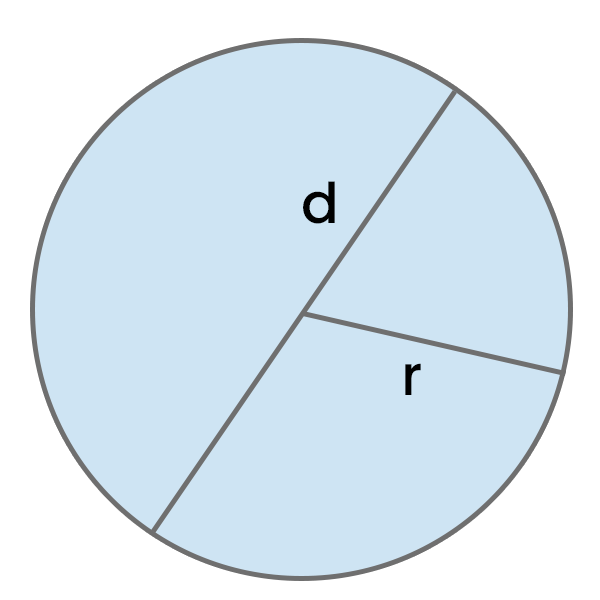# Circumference of a Circle Calculator

Calculate the circumference of a circle based on the provided input data (radius or diameter) and see the detailed process of calculations.

c = 2π * rResult:

## How to calculate Circumference of a Circle?

To calculate the circumference of a circle, use the formula:
Circumference = 2 * π * radius
Plug in the value of the radius and the constant π (approximately 3.14) into the formula to obtain the circle's circumference. If you want to check the value of PI for a specific number of decimal places, see the PI Digits calculator.
Example: Calculate the circumference of a circle with a radius of 10 cm.

C = 2 * π * 10 cm

C ≈ 2 * 3.14 * 10 cm

C ≈ 6.28 * 10 cm

C ≈ 62.8 cm

## How to use this calculator?

To use this calculator, input the radius or diameter of the circle (fill in one, and the other will be automatically calculated) in your chosen units.

After entering this data, proceed to the results section and select the unit. You can also review the detailed calculation process and see the area of the circle.